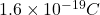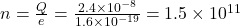## Air “breaks down” when the electric field strength reaches 3 × 106 N/C, causing a spark. A parallel-plate capacitor is made from two 3.0 cm

Question

Air “breaks down” when the electric field strength reaches 3 × 106 N/C, causing a spark. A parallel-plate capacitor is made from two 3.0 cm × 3.0 cm electrodes.How many electrons must be transferred from one electrode to the other to create a spark between the electrodes?

in progress 0
2 weeks 2021-09-02T06:21:44+00:00 1 Answers 0 views 0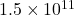Explanation:

We are given that

Electric field=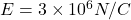Dimension of parallel plate capacitor=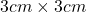Area of parallel plate capacitor=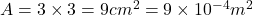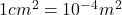We have to find the number of electrons must be transferred from one electrode to the other to create  a spark between the electrodes.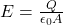Where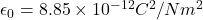Substitute the values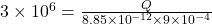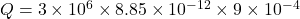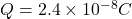We know that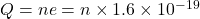Where e=